# RD Sharma Class 9 and Chapter Measures Of Central Tendency Maths Solutions

## Maths Class 9 and Chapter Measures Of Central Tendency RD Sharma SolutionsFilter
Filters :
CLASSES
•
•
•
•
•
•
•
CHAPTERS
•
• 15 More

The previous two units, that is, chapter 22 and chapter 23 from RD Sharma’s textbook, purely dealt with the concept of statistics. These chapters neatly demonstrated the different methods to represent and interpret the given data. The tabular representation of data and different graphical means of expressing the data is found to be widely applicable in various fields. Now the next part of the statistics would be the Measures of Central Tendency, that forms the chapter 24 of class 9 RD Sharma Maths book.

Measures of central tendency’ is nothing, but measuring the central value of a particular data set. This average value is a number through the means of which the whole distribution of data can be interpreted. Measures of central tendency involve the usage of terms such as mean, meridian, and mode. Mean, meridian, and mode are the measures of central tendency, but the appropriate measure to be chosen depends upon the given conditions.

## What is the mean?

The mean is also called as the ‘average.’ The mean or average of a number set will be the summation of the given data/values, divided by the total number of values. According to the textbook, the ‘mean’ is defined as, “The mean (or average) of a number of observations is the sum of the values of all the observations divided by the total number of observations.” The ‘mean,’ is called as ‘x bar’ and it is denoted by the expression, x.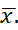Take an example of the data set: 1,3,4,5,7,8,7. The mean of this data would be (1+3+4+5+7+8+7)/7 = 35/5 = 7.

## What is the median?

A median divides the set of given numbers into two parts. Median marks the middle value of the set of data that are arranged in an order of their magnitudes (either ascending or descending).

Let’s find out the median of the given data set. Arranging in terms of their magnitudes, 1,3,4,5,7,7,8. The middle terms or the median would be the number 5 here.

## What is the mode?

The mode is the data value that is said to appear more frequently in a data set. This easily helps to find out the most recurring values or data. The mode is an important parameter that is used widely in manufacturing and other industries for analysing the particular product to be produced in large quantity. The mode, in the above data set, is number 7 as it is repeated twice.

So, this unit, RD Sharma Class 9 Maths Chapter 24 Measures of Central Tendency, is all about the calculation of the mean, median and mode of a given set of data. One can find formulae on these calculations. All these formulae and calculation techniques are well-covered in RD Sharma’s textbook. RD Sharma’s textbooks have been a boon to the CBSE board students for many years now. All the concepts are dealt with much clarity that the students find it easy to understand from the books. Mr Sharma, a Maths professor, has been authoring Maths books for 30 years and has thus mastered the technique of presenting the concepts in a student-friendly way. Many students own RD Sharma’s books despite the NCERT prescribed books being available. This is because referring to RD Sharma’s don’t just help to face the school exams but also serve sufficient to crack the tough entrance exams like the JEE, after school. Mr Sharma’s books help to build the basics strong by providing ample solved problems and practise problems. These practise problems are given in the textbook in the form of exercises and the students can work out these on their own.

RD Sharma’s books have already proved to be one of the best sellers in the Maths textbook category, in India. But for a concept-based subject like Maths, the students owning a relevant solution guide for the chosen reference textbook is also equally important. This helps them in learning a lesson quicker and easier. In this solutions platform category comes Doubtnut, a premium online learning platform where one can find video solutions for the textbooks. The solutions to this chapter are found on Doubtnut as RD Sharma Class 9 Solutions Chapter 24 Measures of Central Tendency.

Why Doubtnut for this chapter, Measures of Central Tendency?

Doubtnut stands out as one of the premium online tutorials platforms where the students can find all the textbook solutions in the form of videos. The textbook solutions are curated by a team of talented subject matter experts and have been presented in an easily understandable way. These video tutorials can be easily accessed by students through the means of their phones or any handheld devices. Apart from video solutions for all textbooks like RD Sharma, RS Agarwal, etc, one can also find NCERT solutions on Doubtnut. Not just the video solutions, one can also find the PDF format of the NCERT solutions that can be downloaded and referred to at any convenient timing.  The students can also get any doubts and questions cleared through the means of Doubtnut website or the app. The queries or questions can be clicked as a picture and uploaded on the platform. These questions will be answered by the expert teachers instantaneously.

## Topics and Subtopics of RD Sharma Class 9 Maths Chapter 24 Measures of Central Tendency

The topics and subtopics of a chapter give the bigger picture of a whole unit. It is always essential to understand the topics and subtopics that are covered under a particular unit. The topics and subtopics give an idea about the entire chapter and this knowledge help the learners to plan the lesson well in advance. Now it is apt to know the breakdown of the content of the chapter in the form of topics and subtopics.

The topics and subtopics of RD Sharma Class 9 Maths Chapter 24, according to which the solutions are worked-out on Doubtnut:

### Introduction to the unit

The importance of knowing the measures of the central tendency instead of all the data is mentioned here.

### Mean, median and mode

A) The mean of a set of data is formulated as,                                                                                                                                                                                        Mean = Sum of all the observations / Total number of observations.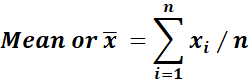Where,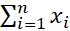is the total of the ‘n’ number of data values/observations, and ‘n’ is the number of observations.

B) The ‘median’ of a given set of data is calculated by two formulae depending upon the number of given values/observations being an even or an odd number.

Thus, the formula for calculating the median for a given data set when the number of observations is odd will be,

The Median =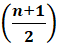th term.

If the median is to be found out for an even set of data, the formula goes as,

The Median = Mean values of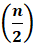th and theth term

C) Mode: Mode is the most frequently occurring observation or value in a set of data given.

All these are the important formulae based on which the problems from this unit are found in the form of exercises in RD Sharma’s book. The problems demand the students tactically apply the formulae in order to solve them. The methodical way to solve such problems is demonstrated on Doubtnut as video solutions. Learning through the videos serves as the premium learning methodology since the learners would be able to retain the learnt lessons better. The language used to explain the concepts is very simple so that it can be understood easily by the students, thus making it more student-friendly. The simple language usage and the immersive learning technology, together that help users learn the lessons effortlessly have made Doubtnut a highly trusted online tutorial medium in recent times. Also, since Doubtnut gives way to clear the doubts and queries easily leads to swift learning of the lessons. These video tutorials can be accessed by the students all over the country irrespective of their financial situations. This is because these video solutions are available free of cost, and thus, the students who cannot afford to attend tutorial classes immensely benefit from Doubtnut.

Share this on :-